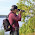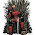## Monday, February 4, 2013

### MOJO Wins NFL Picks Contest!

The Ravens weren't the only ones to hold on for a big win on Sunday. Memphis MOJO was able to hold off Vegas Vic and TwoMinuteWarning to win the NFL Picks Contest.

Congratulations MOJO! Well done!

lightning- No pick = 0 pts.
TwoMinuteWarning- Baltimore (W 34-31) = 13 pts.
Grange95- San Francisco (L 31-34) = <3> pts.
Music City Momma- San Francisco (L 31-34) = <3> pts.
Memphis MOJO-  Baltimore (W 34-31) = 13 pts.
Vegas Vic-  San Francisco (L 31-34) = <3> pts.
Captain Crunch- San Francisco (L 31-34) = <3> pts.

Here are the final standings;

Memphis MOJO - 40+19+22+13+16+18+24+68+22+17+45+24-(3)+14+13 = 352 pts.
TwoMinuteWarning - 13+19+22+16+31+18+19+23+31+58+24+24+23-(15)+13 =319
Vegas Vic - 16+19+22+13+16+40+15+23+43+58+24+24+23-(15)-(3) = 318
lightning - 13+19+24+13+16+18+24+24+22+17+38+24+23+14+0 =289
Music City Momma - 17+19+24+5+17+34+24+24+43+17+24+24+23-(15)-(3) = 277
Grange95 - 20+19+22+13+14+40+24+23+43+17+45-(15)-(3)+14-(3) = 273
Captain Crunch - 13+11+18+16+31+18+24-(2)+31+32+24+20+23-(4)-(3) =252

Thank you to everyone who participated. It was a lot of fun and I'll definitely do it again next season!

1.2.